# 前言

1. 樹和二叉樹的基本知識（這你總得會吧）
2. 二叉堆（主要是堆式儲存）
3. 離散化（其實并不需要）
4. 會寫代碼

# 基礎線段樹

## 線段樹入門

$\left \{ 1,1,4,5,1,4 \right \}$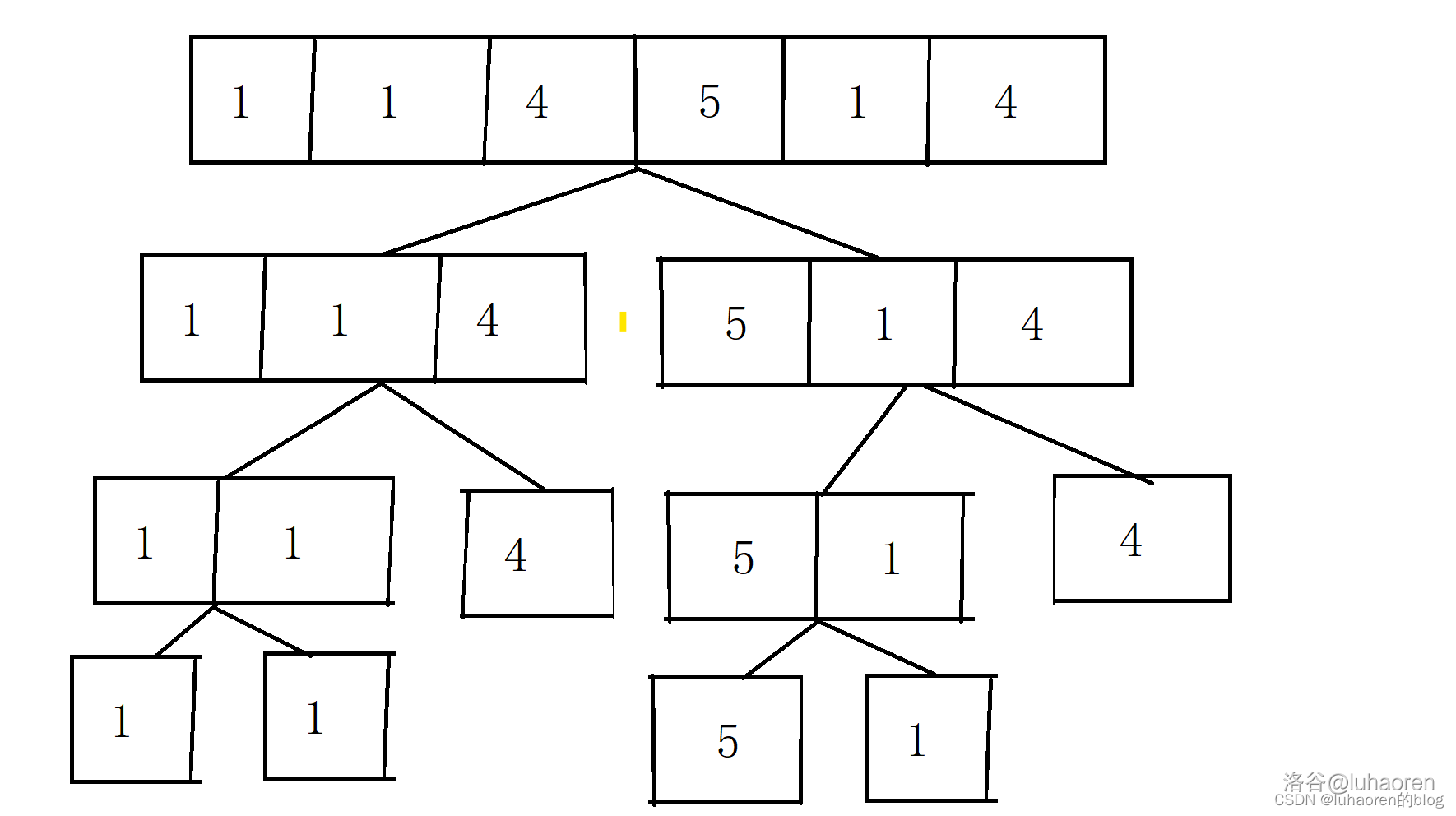void pushup(int x){
tr[x].sum=tr[x*2].sum+tr[x*2+1].sum;
}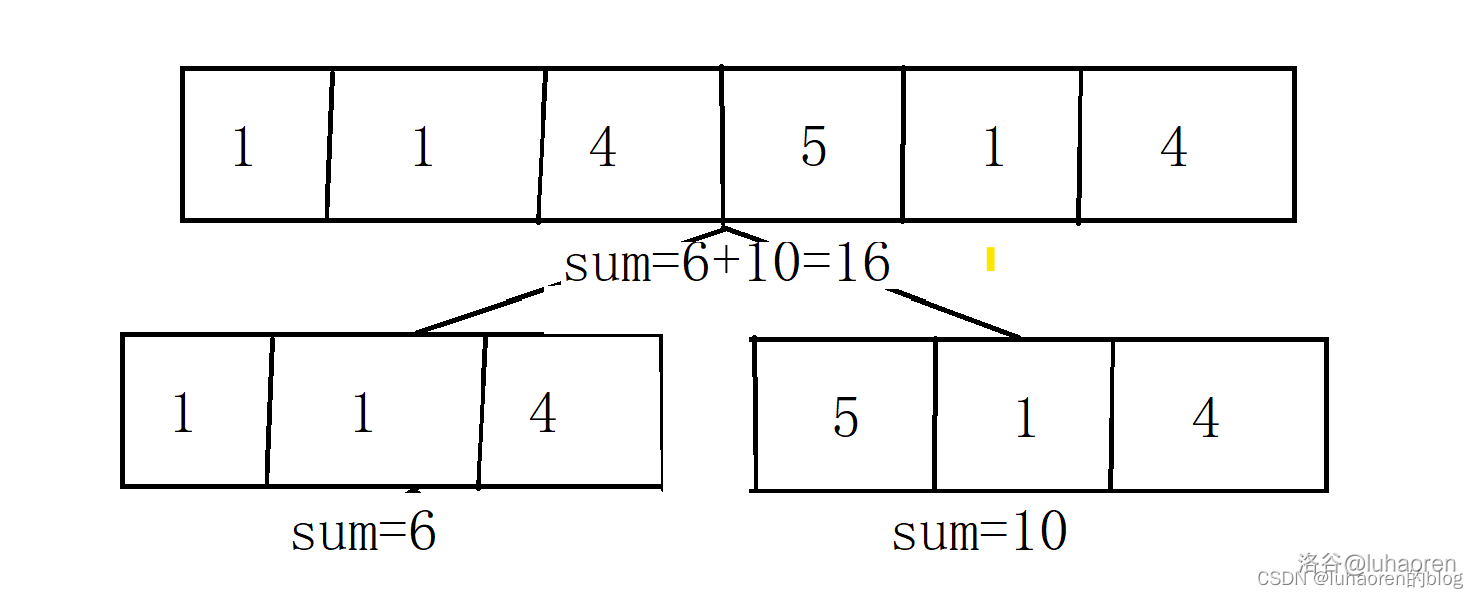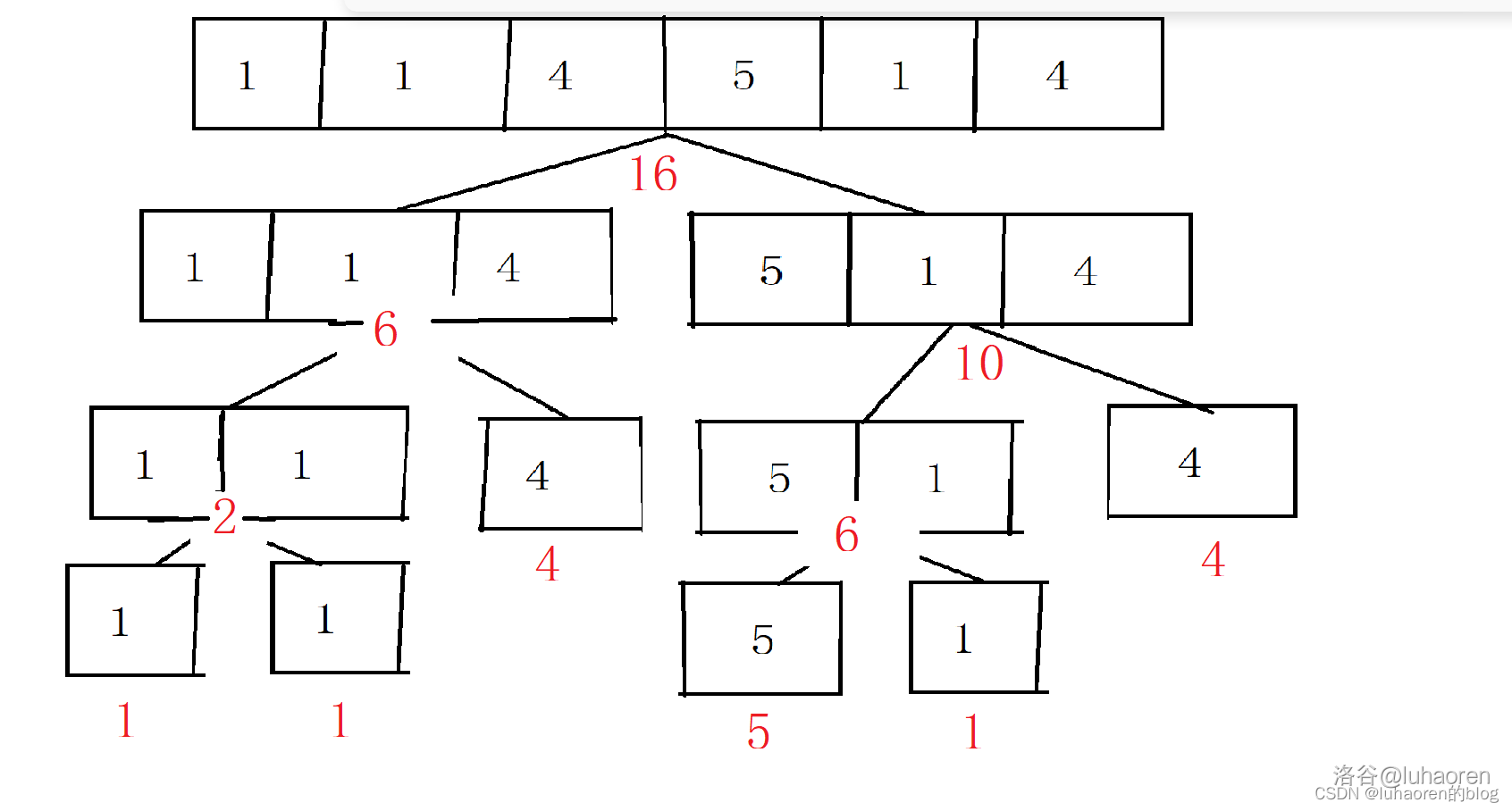void build(int x,int l,int r){
tr[x].l=l,tr[x].r=r;//節點表示區間的左右界
if(l==r){
//若l=r，說明這個節點是葉子節點，直接賦值
tr[x].sum=a[l];//a是原數列
return;
}
int mid=(l+r)/2;//mid表示左右子區間的間隔
build(x*2,l,mid),build(x*2+1,mid+1,r);//遞歸建樹
//線段樹是完全二叉樹，左右子節點可以用x*2,x*2+1表示
tr[x].sum=tr[x*2].sum+tr[x*2+1].sum;//pushup操作
}


### 區間查詢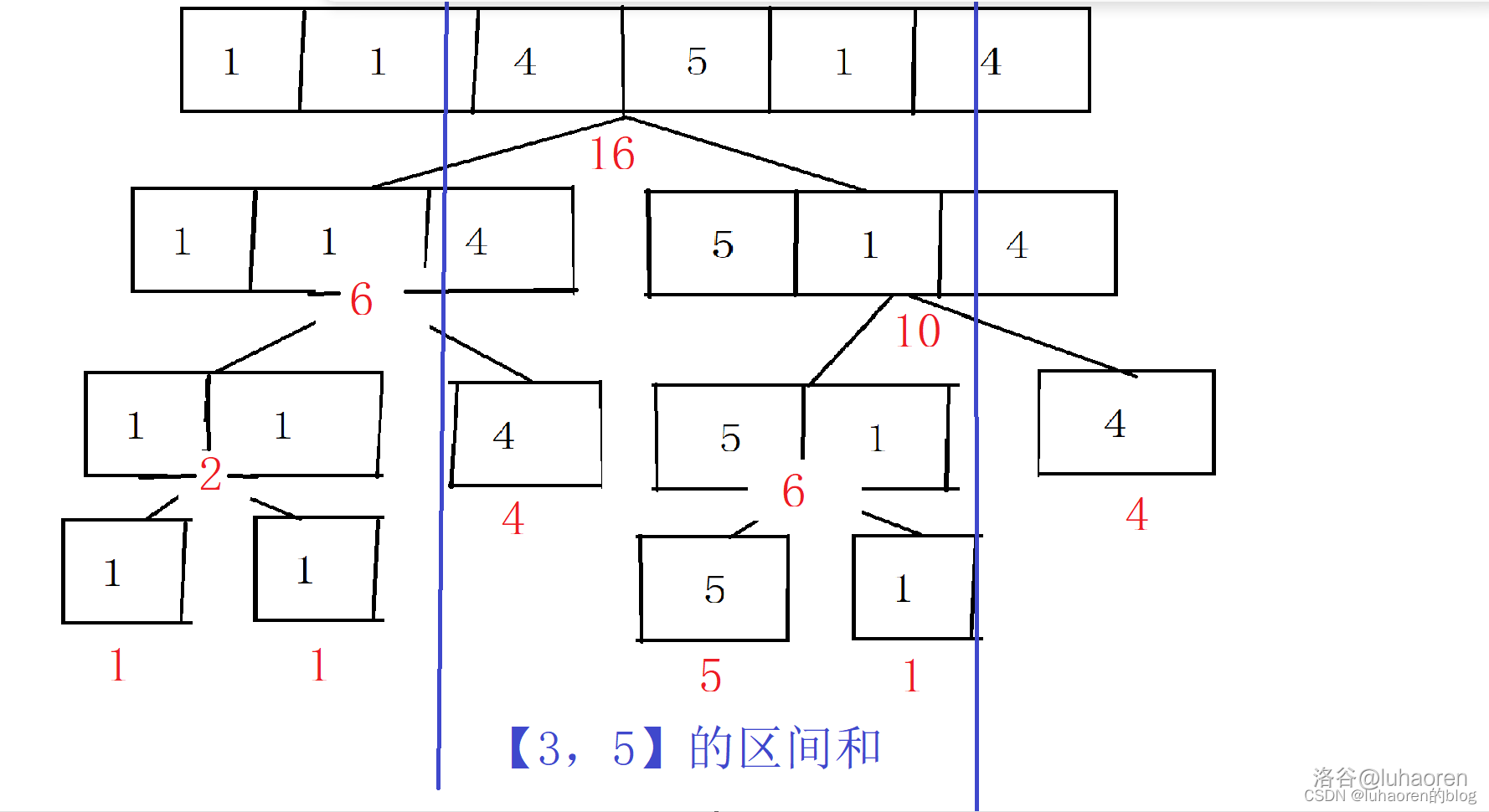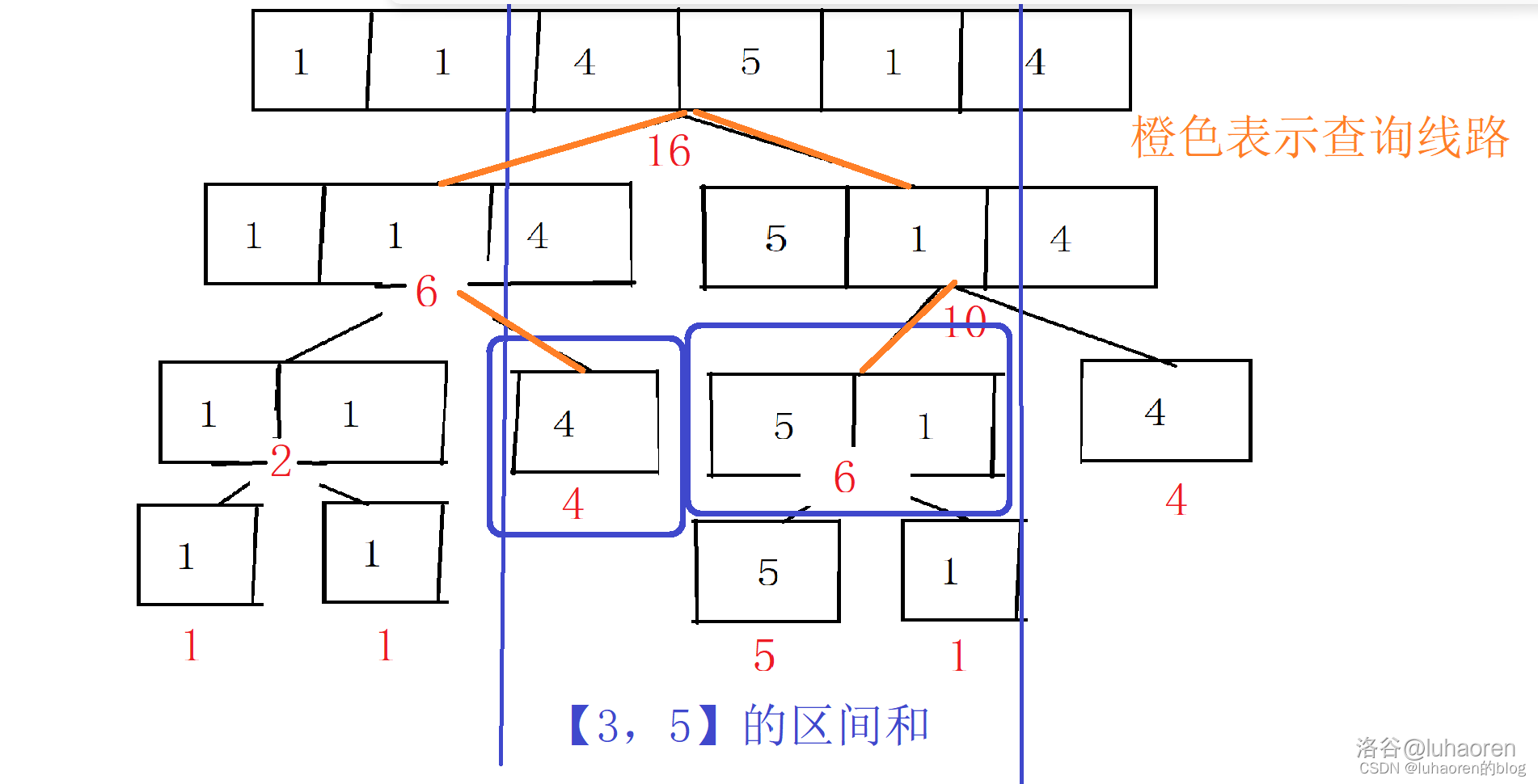int query(int x,int l,int r){
//區間查詢
if(tr[x].l>=l&&tr[x].r<=r) return tr[x].sum;//如果該節點的區間被要查找的區間包括了，那么就不用繼續找了，直接返回改節點的值就行了
int mid=(tr[x].l+tr[x].r)/2;
int sum=0;
if(l<=mid) sum+=query(x*2,l,r);//如果當前節點在要查找區間左邊界的右面，那么遞歸查找左子樹
if(r>mid) sum+=query(x*2+1,l,r);//如果當前節點在要查找區間右邊界的左面，那么遞歸查找右子樹
return sum;//由此得出了該區間的值，返回即可
}


### 單點修改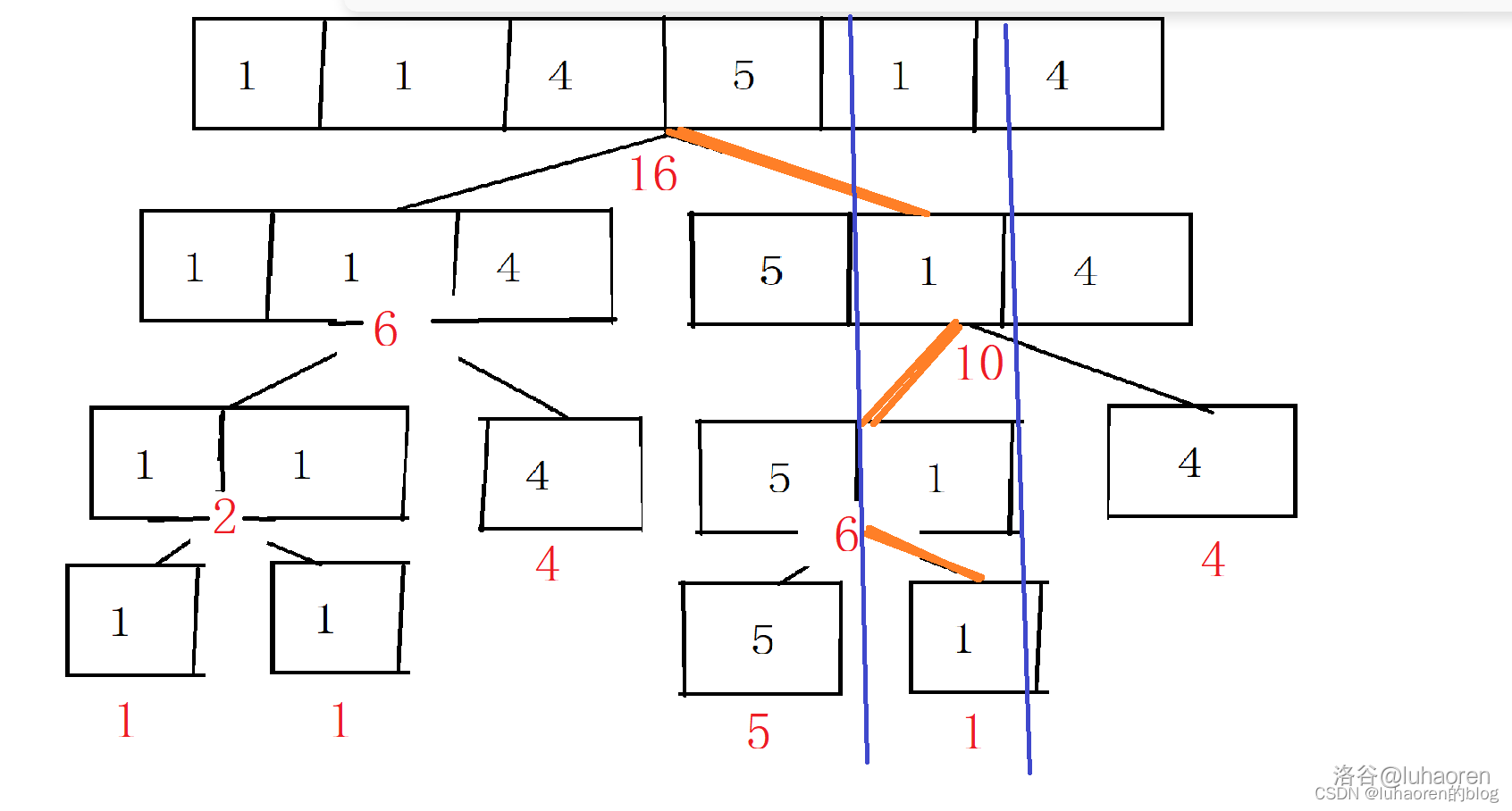void change(int now,int x,int k){
//單點修改
if(tr[now].l==tr[now].r){
tr[now].sum=k;//如果找到了該節點，那么修改它
return;
}
int mid=(tr[now].l+tr[now].r)/2;
if(x<=mid) change(now*2,x,k);//如果要尋找的節點在當前節點的左側，就遞歸左子樹
else change(now*2+1,x,k);//否則遞歸右子樹
tr[now].sum=tr[now*2].sum+tr[now*2+1].sum;//pushup操作，維護每個節點的sum值
}


### 線段樹的存儲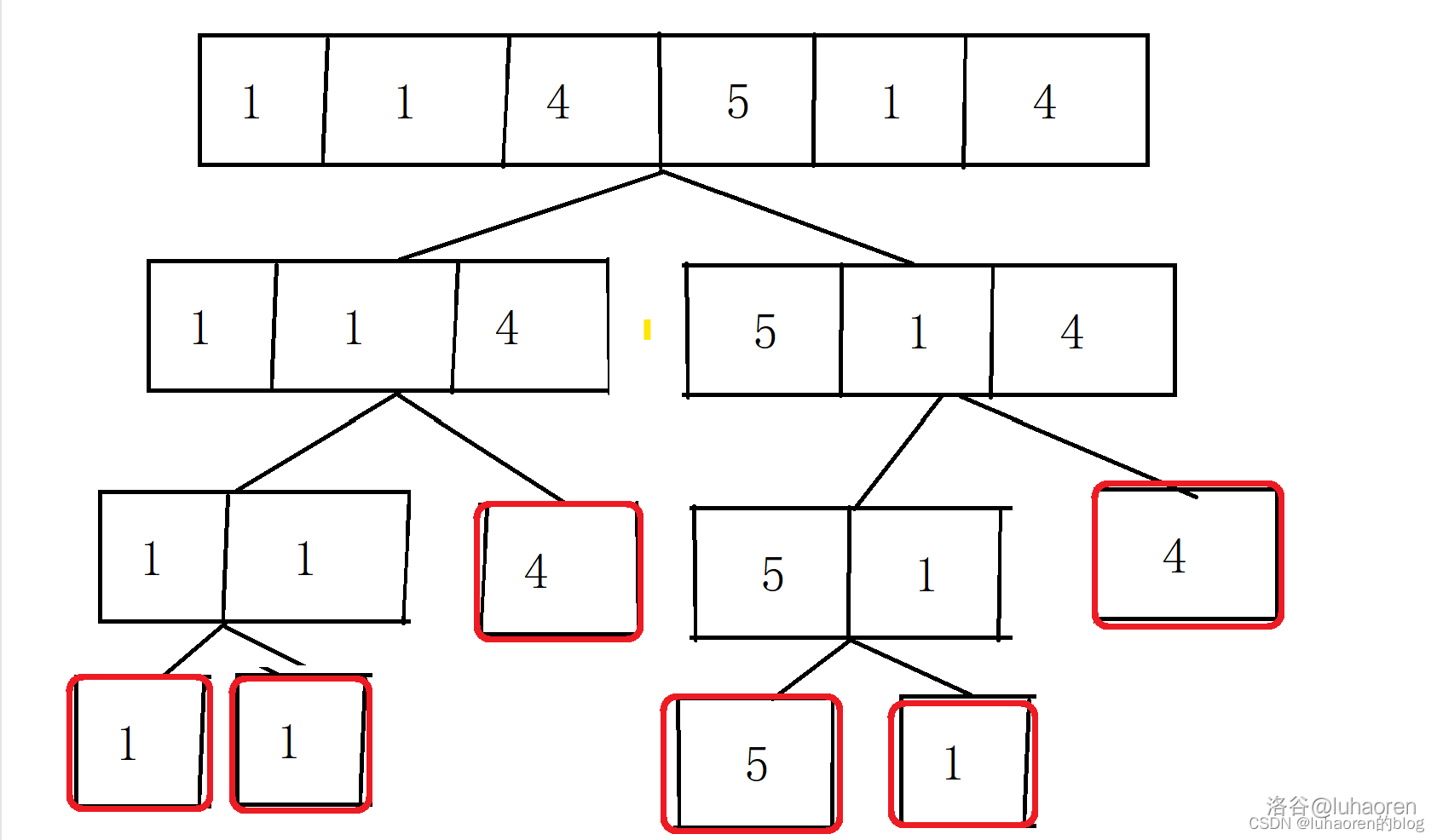### 例題1：單點修改，區間查詢

• 將某一個數加上$$x$$
• 求出某區間每一個數的和

AC代碼

#include <bits/stdc++.h>
using namespace std;
const int N=1e6+10;
struct node{
int sum,l,r;//線段樹節點的結構體
}tr[N*4];//線段樹需要開四倍空間
int a[N];
inline void pushup(int x){
tr[x].sum=tr[x*2].sum+tr[x*2+1].sum;
}
void build(int x,int l,int r){
tr[x].l=l,tr[x].r=r;//節點表示區間的左右界
if(l==r){
//若l=r，說明這個節點是葉子節點，直接賦值
tr[x].sum=a[l];//a是原數列
return;
}
int mid=(l+r)/2;//mid表示左右子區間的間隔
build(x*2,l,mid),build(x*2+1,mid+1,r);//遞歸建樹
//線段樹是完全二叉樹，左右子節點可以用x*2,x*2+1表示
pushup(x);//pushup操作
}
int query(int x,int l,int r){
//區間查詢
if(tr[x].l>=l&&tr[x].r<=r) return tr[x].sum;//如果該節點的區間被要查找的區間包括了，那么就不用繼續找了，直接返回改節點的值就行了
int mid=(tr[x].l+tr[x].r)/2;
int sum=0;
if(l<=mid) sum+=query(x*2,l,r);//如果當前節點在要查找區間左邊界的右面，那么遞歸查找左子樹
if(r>mid) sum+=query(x*2+1,l,r);//如果當前節點在要查找區間右邊界的左面，那么遞歸查找右子樹
return sum;//由此得出了該區間的值，返回即可
}
void change(int now,int x,int k){
//單點修改
if(tr[now].l==tr[now].r){
tr[now].sum+=k;//如果找到了該節點，那么修改它
return;
}
int mid=(tr[now].l+tr[now].r)/2;
if(x<=mid) change(now*2,x,k);//如果要尋找的節點在當前節點的左側，就遞歸左子樹
else change(now*2+1,x,k);//否則遞歸右子樹
pushup(now);//pushup操作，維護每個節點的sum值
}

int n,q;
int main(){
cin>>n>>q;
for(int i=1;i<=n;i++) cin>>a[i];
build(1,1,n);//建樹
while(q--){
int t,b,c;
cin>>t>>b>>c;
if(t==1) change(1,b,c);
else cout<<query(1,b,c)<<endl;
}
}


### 習題

1. JSOI2008 最大數
線段樹維護最大值的模板
2. loj10123. Balanced Lineup
RMQ問題，可以試試用線段樹做

## 懶標記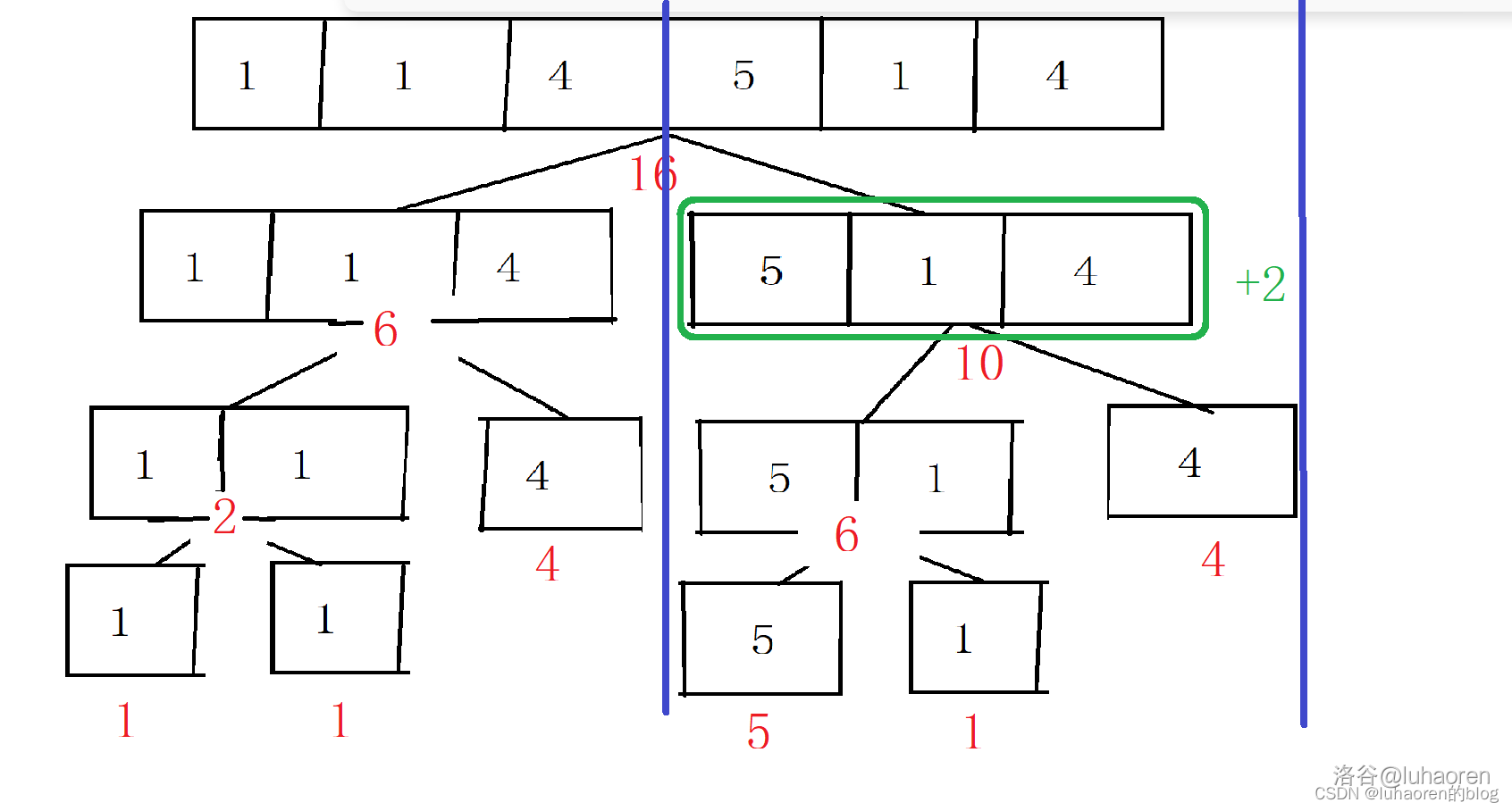### pushdown操作

pushdown代碼

inline void pushudown(int x){
//如果這個節點上有懶標記
//把這個節點的懶標記給他的兩個子節點
//分別給它的兩個子節點修改
//別忘了清空這個節點的懶標記
}
}


### 區間修改

void update(int now,int l,int r,int k){
if(l<=tr[now].l&&r>=tr[now].r){
//如果查到子區間了
tr[now].sum+=k*(tr[now].r-tr[now].l+1);//先修改這個區間
//注：這里一定要分清順序，先修改，再標記！
}
else{
//如果需要繼續向下查詢
pushudown(now);//一定要先把懶標記向下傳
int mid=(tr[now].l+tr[now].r)/2;
//這里很像區間查詢
if(l<=mid) update(now*2,l,r,k);
if(r>mid) update(now*2+1,l,r,k);
//最后別忘了pushup一下
pushup(now);
}
}


### 例題2：區間修改，區間查詢

1. 將某區間每一個數加上$$k$$。
2. 求出某區間每一個數的和。

AC代碼

#include <bits/stdc++.h>
using namespace std;
const int N=1e5+10;
struct node{
int sum;
int l,r;
}tr[N*4];//線段樹要開四倍空間哦
int a[N];//原數列
inline void pushup(int x){
tr[x].sum=tr[2*x].sum+tr[2*x+1].sum;//pushup操作
}
inline void pushudown(int x){
//如果這個節點上有懶標記
//把這個節點的懶標記給他的兩個子節點
//分別給它的兩個子節點修改
//別忘了清空這個節點的懶標記
}
}
void build(int x,int l,int r){
//建樹操作
if(l==r){
tr[x].sum=a[l];
return;
}
int mid=(l+r)/2;
build(2*x,l,mid),build(2*x+1,mid+1,r);
pushup(x);
}
int query(int x,int l,int r){
if(l<=tr[x].l&&r>=tr[x].r) return tr[x].sum;
pushudown(x);//注意，區間查詢時也要下懶傳標記
int mid=(tr[x].l+tr[x].r)/2,sum=0;
if(l<=mid) sum+=query(x*2,l,r);
if(r>mid) sum+=query(x*2+1,l,r);
return sum;
}
void update(int now,int l,int r,int k){
if(l<=tr[now].l&&r>=tr[now].r){
//如果查到子區間了
tr[now].sum+=k*(tr[now].r-tr[now].l+1);//先修改這個區間
//注：這里一定要分清順序，先修改，再標記！
}
else{
//如果需要繼續向下查詢
pushudown(now);//先把懶標記向下傳
int mid=(tr[now].l+tr[now].r)/2;
//這里很像區間查詢
if(l<=mid) update(now*2,l,r,k);
if(r>mid) update(now*2+1,l,r,k);
pushup(now);//最后別忘了pushup一下
}
}
int n,q;
int main(){
cin>>n>>q;
for(int i=1;i<=n;i++) cin>>a[i];
build(1,1,n);
while(q--){
int l,r,k,c;
cin>>c>>l>>r;
if(c==1){
cin>>k;
update(1,l,r,k);
}
else cout<<query(1,l,r)<<endl;
}
return 0;
}
//別忘了開long long哦


### 例題3：較復雜的區間操作

1. 將某區間每一個數乘上$$x$$。

2. 將某區間每一個數加上$$x$$。

3. 求出某區間每一個數的和。

AC代碼

#include <bits/stdc++.h>
using namespace std;
const int N=1e5+10;
struct node{
int l,r;
}tr[N*4];//線段樹開四倍空間
int a[N];
int n,p,m;
inline void pushup(int x){
tr[x].sum=(tr[2*x].sum+tr[2*x+1].sum)%p;
}
inline void eval(int x,int add,int mul){
//我們把計算懶標記單獨放在這個函數里，否則好多東西擠一塊很難看
tr[x].mul=(mul*tr[x].mul)%p; 	//先計算乘法懶標記
}

inline void pushdown(int x){
//依次計算兩個子節點的值和懶標記
//清空懶標記，注意：乘法懶標記要初始化成1
}
void build(int x,int l,int r){
tr[x].l=l,tr[x].r=r;
if(l==r){
tr[x].sum=a[l];
return;
}
int mid=(l+r)/2;
build(x*2,l,mid),build(x*2+1,mid+1,r);//遞歸建樹
pushup(x);
}
void change(int x,int l,int r,int add,int mul){
else{
pushdown(x);
int mid=(tr[x].l+tr[x].r)/2;
pushup(x);
}
}
int query(int x,int l,int r){
if(l<=tr[x].l&&r>=tr[x].r) return tr[x].sum;
int sum=0;
pushdown(x); //區間查詢時也要pushdown
int mid=(tr[x].l+tr[x].r)/2;
if(l<=mid) sum+=query(x*2,l,r)%p;
if(r>mid) sum+=query(x*2+1,l,r)%p;
return sum;
}
int main(){
int t,g,c,ch;
cin>>n>>m>>p;
for(int i=1;i<=n;i++) cin>>a[i];
build(1,1,n);
while(m--){
cin>>ch>>t>>g;
if(ch==1){
cin>>c;
change(1,t,g,0,c);
}
else if(ch==2){
cin>>c;
change(1,t,g,c,1);
}
else cout<<query(1,t,g)%p<<endl;
}
return 0;
}
//記得開longlong


### 標記永久化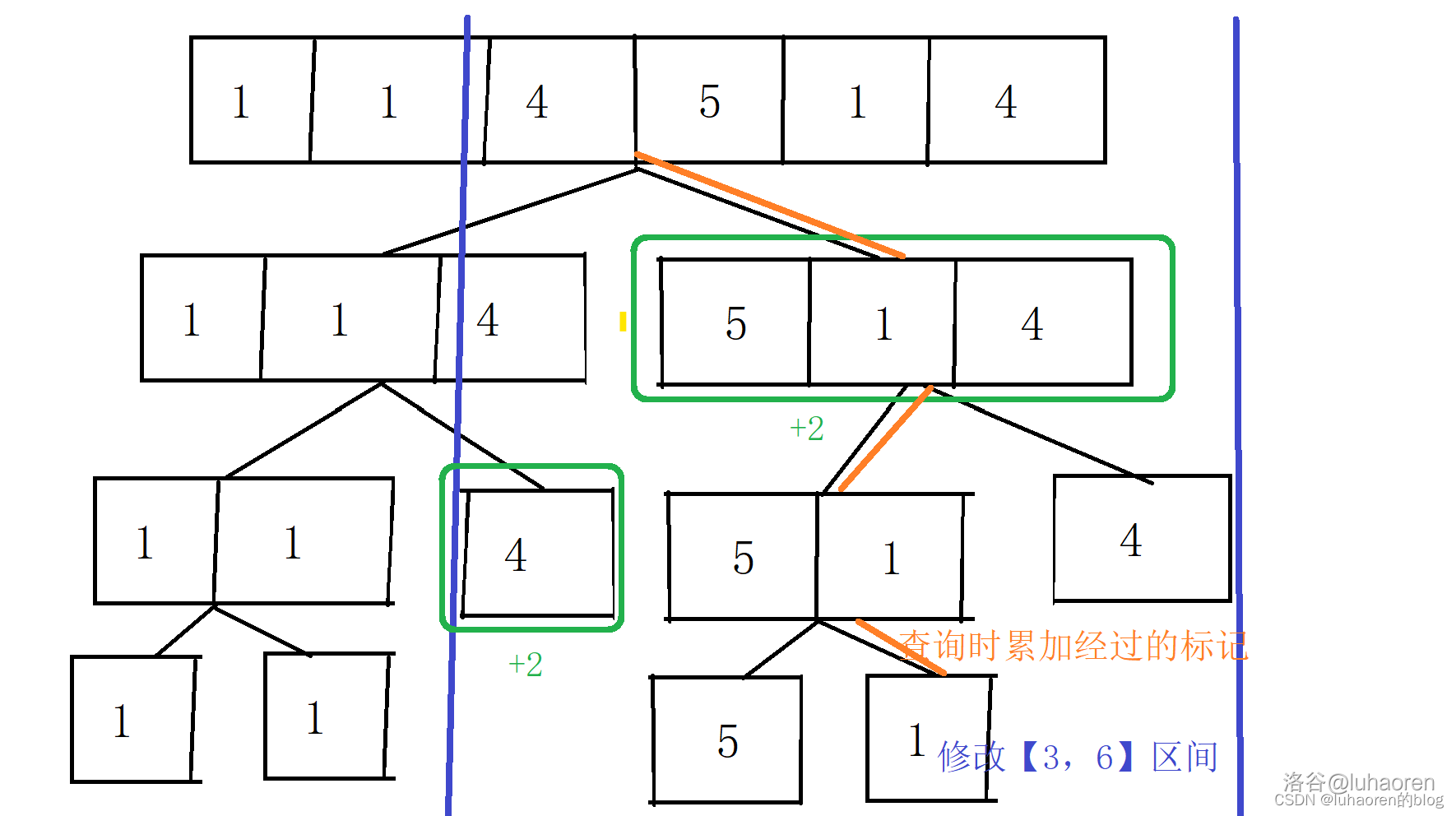const int N=1e4+10;
struct node{
int l,r;
}tr[N*4];
int a[N];
void build(int x,int l,int r){
tr[x].l=l,tr[x].r=r;
if(l==r){
return;
}
int mid=(l+r)/2;
build(x*2,l,mid),build(x*2+1,mid+1,r);
tr[x].sum=tr[x*2].sum+tr[x*2+1].sum;//標記永久化中只有建樹時需要用到pushup操作
}
void update(int x,int l,int r,int k){
tr[x].sum+=(min(tr[x].r,r)-max(tr[x].l,l)+1)*k;//要取一個交集來加
if(tr[x].l>=l&&tr[x].r<=r){
return;
}
int mid=(tr[x].l+tr[x].r)/2;
if(l<=mid) update(x*2,l,r,k);
if(r>mid) update(x*2+1,l,r,k);
}
int query(int x,int l,int r,int add){
if(tr[x].l>=l&&tr[x].r<=r){
return tr[x].sum+s;
}
int mid=(tr[x].l+tr[x].r)/2,sum=0;
return sum;
}


### 習題

1. AHOI2009 維護序列
與例題3差不多
2. 洛谷P1253 扶蘇的問題
稍微復雜的懶標記維護
3. 洛谷P5142 區間方差
需要一定的數學推導能力
4. P4145 花神游歷各國
想一想如何優化？
5. P1471 方差
3題的加強版，較難
6. P6327 區間加區間sin和
需要一些高中的數學知識

# 權值線段樹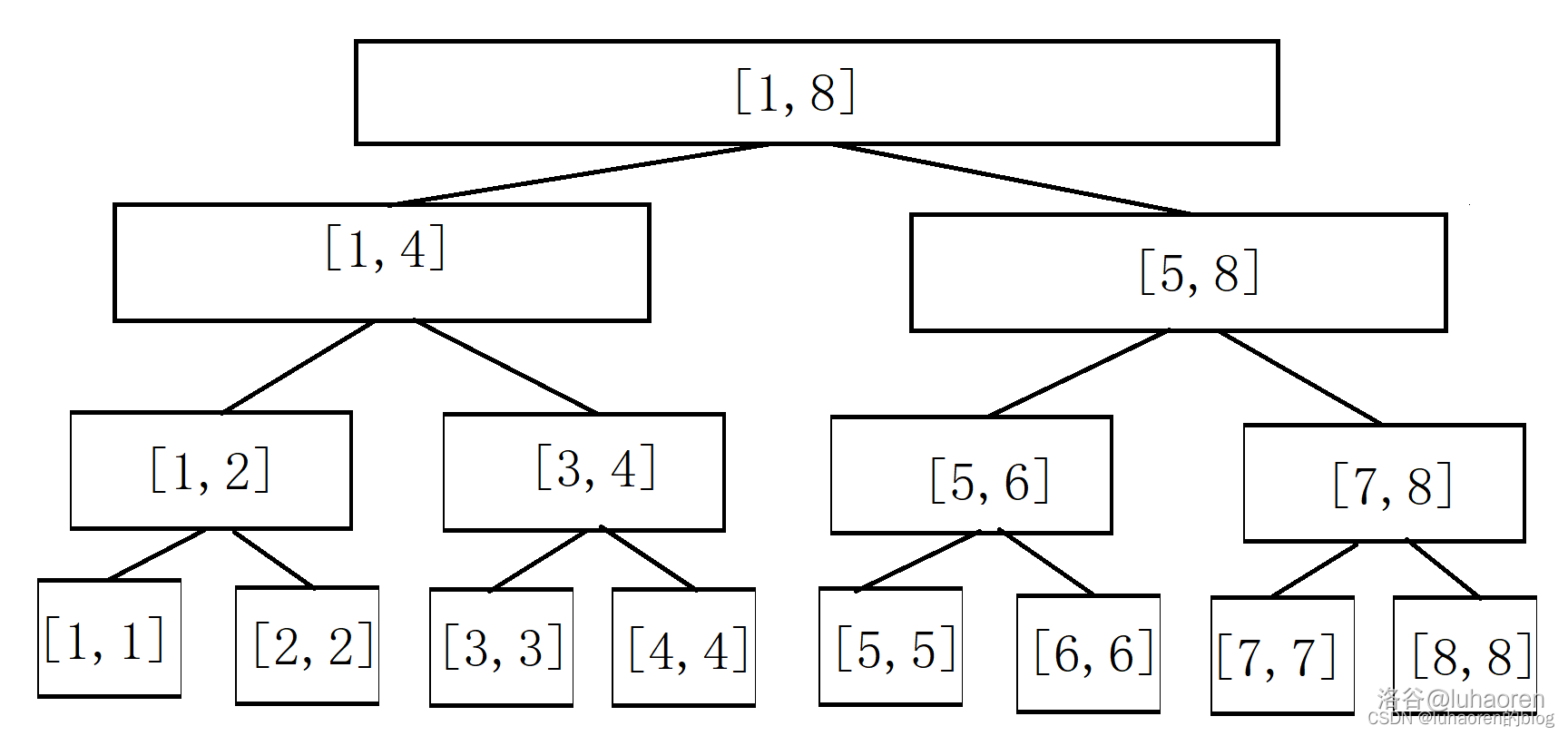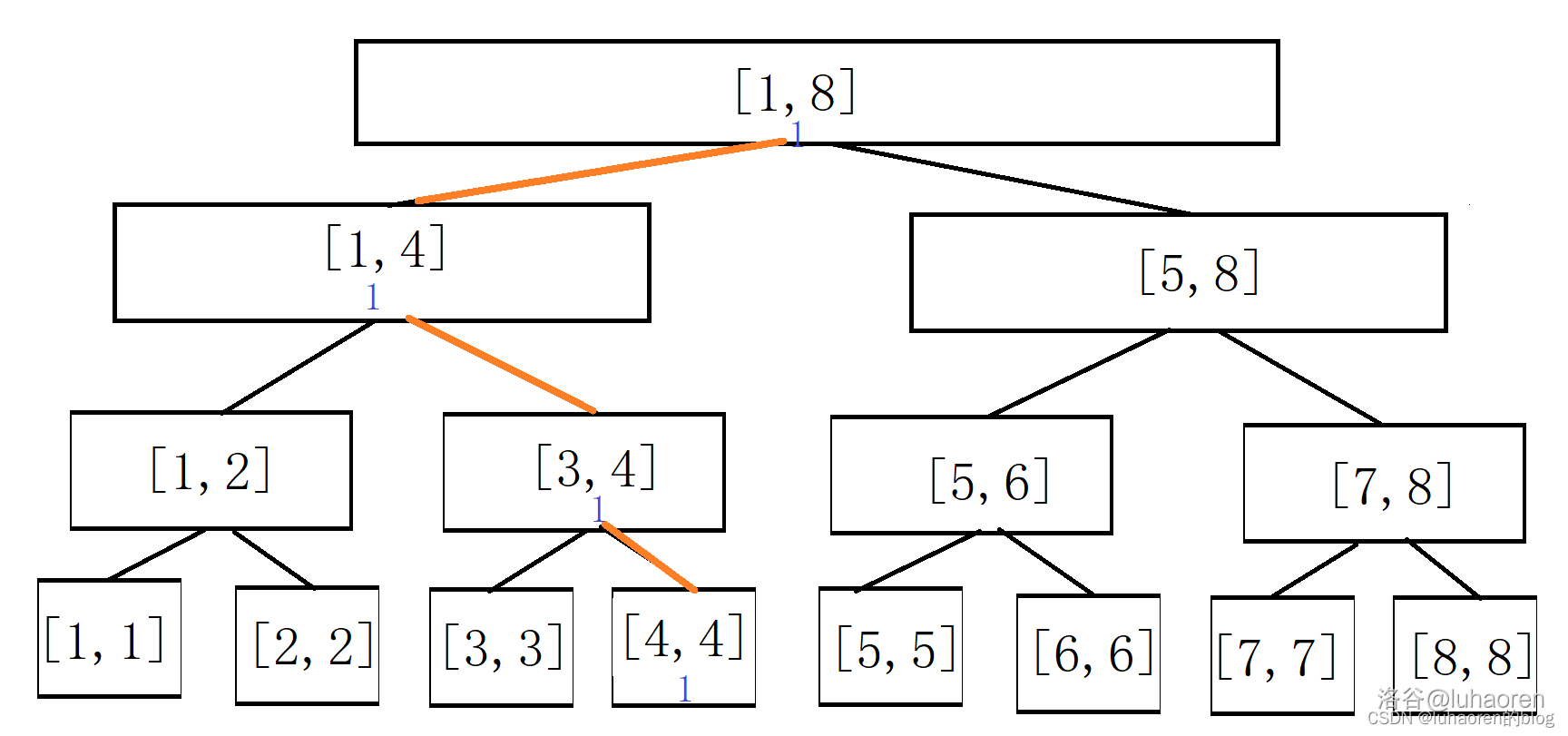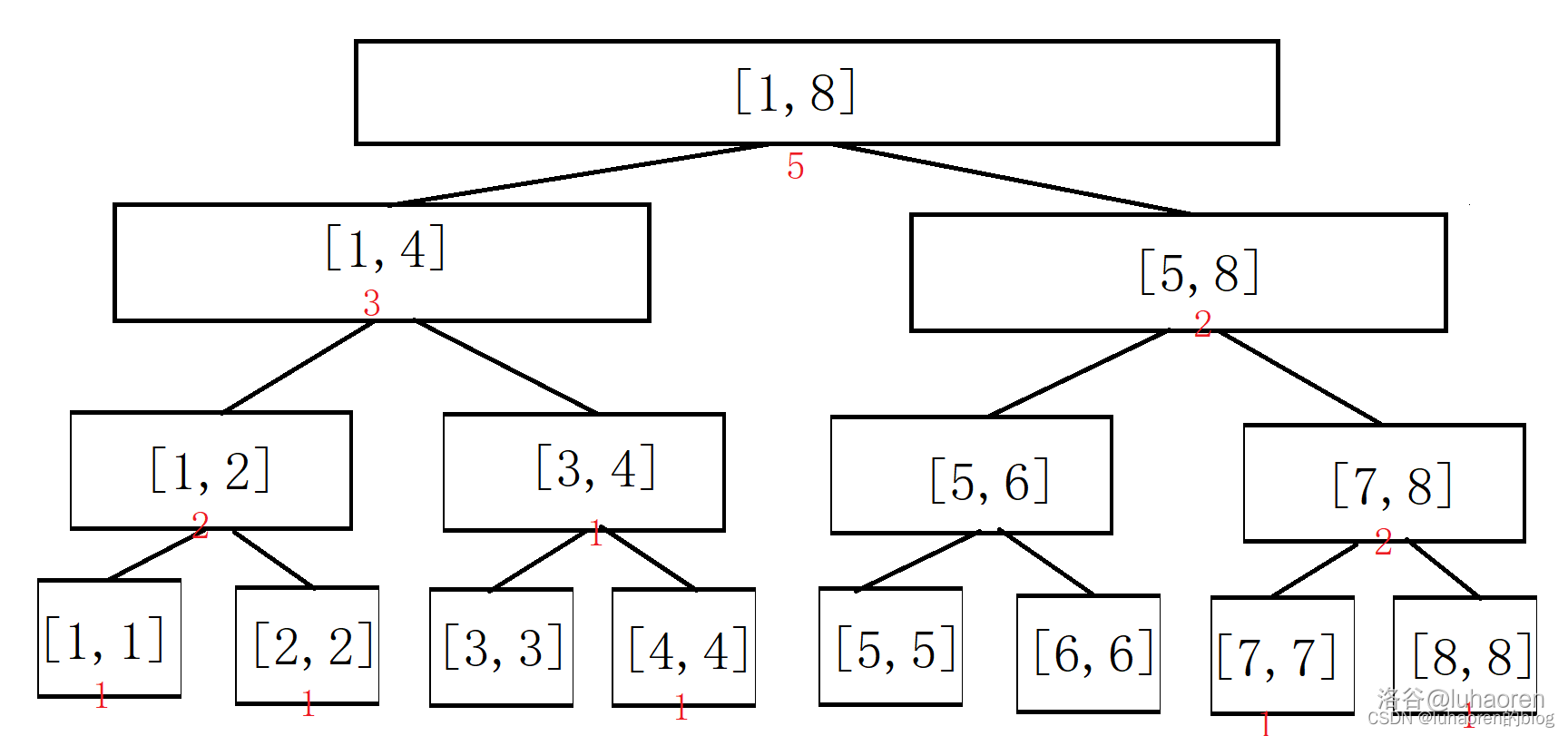### 插入與刪除

int tr[N*4];
//這就是上文提到過的線段樹的另一種寫法，因為權值線段樹不需要維護區間信息，所以不需要建樹的預處理，這種寫法就變得很方便。
inline void pushup(int x){
tr[x]=tr[x*2]+tr[x*2+1];
}
void insert(int x,int l,int r,int k){
//插入一個數k
if(l==r){
tr[x]++;
return;
}
int mid=(l+r)/2;
if(k<=mid) insert(x*2,l,mid,k);
else insert(x*2+1,mid+1,r,k);
pushup(x);
}
void del(int x,int l,int r,int k){
//刪除一個數k
if(l==r){
tr[x]--;
return;
}
int mid=(l+r)/2;
if(k<=mid) del(x*2,l,mid,k);
else del(x*2+1,mid+1,r,k);
pushup(x);
}
int query(int x,int l,int r,int ql,int qr){
//查詢ql，qr之間一共有多少個數
if(l>=ql&&r<=qr) return tr[x];
int mid=(l+r)/2,sum=0;
if(ql<=mid) sum=query(x*2,l,mid,ql,qr);
if(qr>mid) sum+=query(x*2+1,mid+1,r,ql,qr);
return sum;
}


### 例題4：權值線段樹

loj 10116

NK 中學組織同學們去五云山寨參加社會實踐活動，按慣例要乘坐火車去。由于 NK 中學的學生很多，在火車開之前必須清點好人數。

AC代碼

#include <bits/stdc++.h>
using namespace std;
const int N=5e5+10;
int tr[N*4];//權值線段樹維護的是值域，所以要開n的范圍的四倍
inline void pushup(int x){
tr[x]=tr[x*2]+tr[x*2+1];
}
void insert(int x,int l,int r,int k,int p){
if(l==r){
tr[x]+=p;
return;
}
int mid=(l+r)/2;
if(k<=mid) insert(x*2,l,mid,k,p);
else insert(x*2+1,mid+1,r,k,p);
pushup(x);
}
int query(int x,int l,int r,int ql,int qr){
if(l>=ql&&r<=qr) return tr[x];
int mid=(l+r)/2,sum=0;
if(ql<=mid) sum=query(x*2,l,mid,ql,qr);
if(qr>mid) sum+=query(x*2+1,mid+1,r,ql,qr);
return sum;
}
int n,k;
int main(){
cin>>n>>k;
while(k--){
char opt;
int m,p;
cin>>opt;
if(opt=='A'){
cin>>m;
cout<<query(1,1,n,1,m)<<endl;
}
else if(opt=='B'){
//上車
cin>>m>>p;
insert(1,1,n,m,p);
}
else{
//下車
cin>>m>>p;
insert(1,1,n,m,-p);
}
}
}


### 查詢第k大數

int kth(int x,int l,int r,int k){
if(l==r) return l;//查到了，返回即可
int mid=(l+r)/2;
if(k<=tr[x*2]) return kth(x*2,l,mid,k);
return kth(x*2+1,mid+1,r,k-tr[x*2]);
}


### 查詢一個數的排名

int rnk(int x,int l,int r,int k){
if(l==r) return 1;
int mid=(l+r)/2;
if(k<=mid) return rnk(x*2,l,mid,k);
return rnk(x*2+1,mid+1,r,k)+tr[x*2];
}


### 例題5：用權值線段樹實現平衡樹

1. 插入$$x$$
2. 刪除$$x$$數(若有多個相同的數，應只刪除一個)
3. 查詢$$x$$數的排名(排名定義為比當前數小的數的個數$$+1$$)
4. 查詢排名為$$x$$的數
5. $$x$$的前驅(前驅定義為小于$$x$$，且最大的數)
6. $$x$$的后繼(后繼定義為大于$$x$$，且最小的數)

#include <bits/stdc++.h>
using namespace std;
const int N=1e7+10;
int tr[8*N];//因為要維護正負區間，所以開二倍，再加線段樹的四倍空間，就是八倍
inline void pushup(int x){
tr[x]=tr[x*2]+tr[x*2+1];
}
void insert(int x,int l,int r,int k,int p){
//若p為1則插入，若p為-1則刪除
if(l==r){
tr[x]+=p;
return;
}
int mid=(l+r)/2;
if(k<=mid) insert(x*2,l,mid,k,p);
else insert(x*2+1,mid+1,r,k,p);
pushup(x);
}
int kth(int x,int l,int r,int k){
//查詢排名為k的數
if(l==r) return l;
int mid=(l+r)/2;
if(k<=tr[x*2]) return kth(x*2,l,mid,k);
return kth(x*2+1,mid+1,r,k-tr[x*2]);
}
int rnk(int x,int l,int r,int k){
//查找數k的排名
if(l==r) return 1;
int mid=(l+r)/2;
if(k<=mid) return rnk(x*2,l,mid,k);
return rnk(x*2+1,mid+1,r,k)+tr[x*2];
}
int n;
int main(){
cin>>n;
while(n--){
int opt,x;
cin>>opt>>x;
switch(opt){
case 1:
insert(1,-N,N,x,1);//插入
break;
case 2:
insert(1,-N,N,x,-1);//刪除
break;
case 3:
cout<<rnk(1,-N,N,x)<<endl;
break;
case 4:
cout<<kth(1,-N,N,x)<<endl;
break;
case 5:
cout<<kth(1,-N,N,rnk(1,0,N,x)-1)<<endl;
break;
case 6:
cout<<kth(1,-N,N,rnk(1,0,N,x)+1)<<endl;
break;
}
}
}


（這兩種方法都會在下一節介紹到）

‘動態開點代碼

#include <bits/stdc++.h>
using namespace std;
const int N=1e7+10,M=4e5+10;
int tr[M];
int ls[M],rs[M],tot=0;
inline void pushup(int x){
tr[x]=tr[ls[x]]+tr[rs[x]];//動態開點后，就不能用x*2的方式存了，得開lsrs兩個數組（或結構體）
}
void insert(int &x,int l,int r,int k,int p){//x是引用形式，方便傳值
if(!x) x=++tot;//動態開點
//若p為1則插入，若p為-1則刪除
if(l==r){
tr[x]+=p;
return;
}
int mid=(l+r)/2;
if(k<=mid) insert(ls[x],l,mid,k,p);
else insert(rs[x],mid+1,r,k,p);
pushup(x);
}
int kth(int x,int l,int r,int k){
if(l==r) return l;//查到了，返回即可
int mid=(l+r)/2;
if(k<=tr[ls[x]]) return kth(ls[x],l,mid,k);
return kth(rs[x],mid+1,r,k-tr[ls[x]]);
}
int rnk(int x,int l,int r,int k){
if(l==r) return 1;
int mid=(l+r)/2;
if(k<=mid) return rnk(ls[x],l,mid,k);
return rnk(rs[x],mid+1,r,k)+tr[ls[x]];
}
int n,root;
int main(){
cin>>n;
while(n--){
int opt,x;
cin>>opt>>x;
switch(opt){
case 1:
insert(root,-N,N,x,1);//因為動態開點的插入寫成引用形式，所以需要帶進去一個變量
break;
case 2:
insert(root,-N,N,x,-1);//刪除
break;
case 3:
cout<<rnk(root,-N,N,x)<<endl;
break;
case 4:
cout<<kth(root,-N,N,x)<<endl;
break;
case 5:
cout<<kth(root,-N,N,rnk(root,-N,N,x)-1)<<endl;
break;
case 6:
cout<<kth(root,-N,N,rnk(root,-N,N,x+1))<<endl;
break;
}
}
}


## 空間優化技巧

### 動態開點

int tr[M];
int ls[M],rs[M],tot=0;
inline void pushup(int x){
tr[x]=tr[ls[x]]+tr[rs[x]];//動態開點后，就不能用x*2的方式存了，得開lsrs兩個數組（或結構體）
}
void insert(int &x,int l,int r,int k){//x是引用形式，方便傳值
if(!x) x=++tot;//動態開點
if(l==r){
tr[x]++;
return;
}
int mid=(l+r)/2;
if(k<=mid) insert(ls[x],l,mid,k);
else insert(rs[x],mid+1,r,k);
pushup(x);
}


### 習題

1. loj10114.數星星 Stars
權值線段樹，需要用動態開點或離散化的優化
2. P1168 中位數
離散化，然后開權值線段樹維護
3. P2073 送花
可以用權值線段樹做
4. SDOI2014 旅行
樹鏈剖分（如果你會的話），用動態開點維護

# zkw線段樹

zkw線段樹是一種用循環實現的線段樹，比正常的遞歸式線段樹快很多，而且好寫。

## zkw線段樹的引入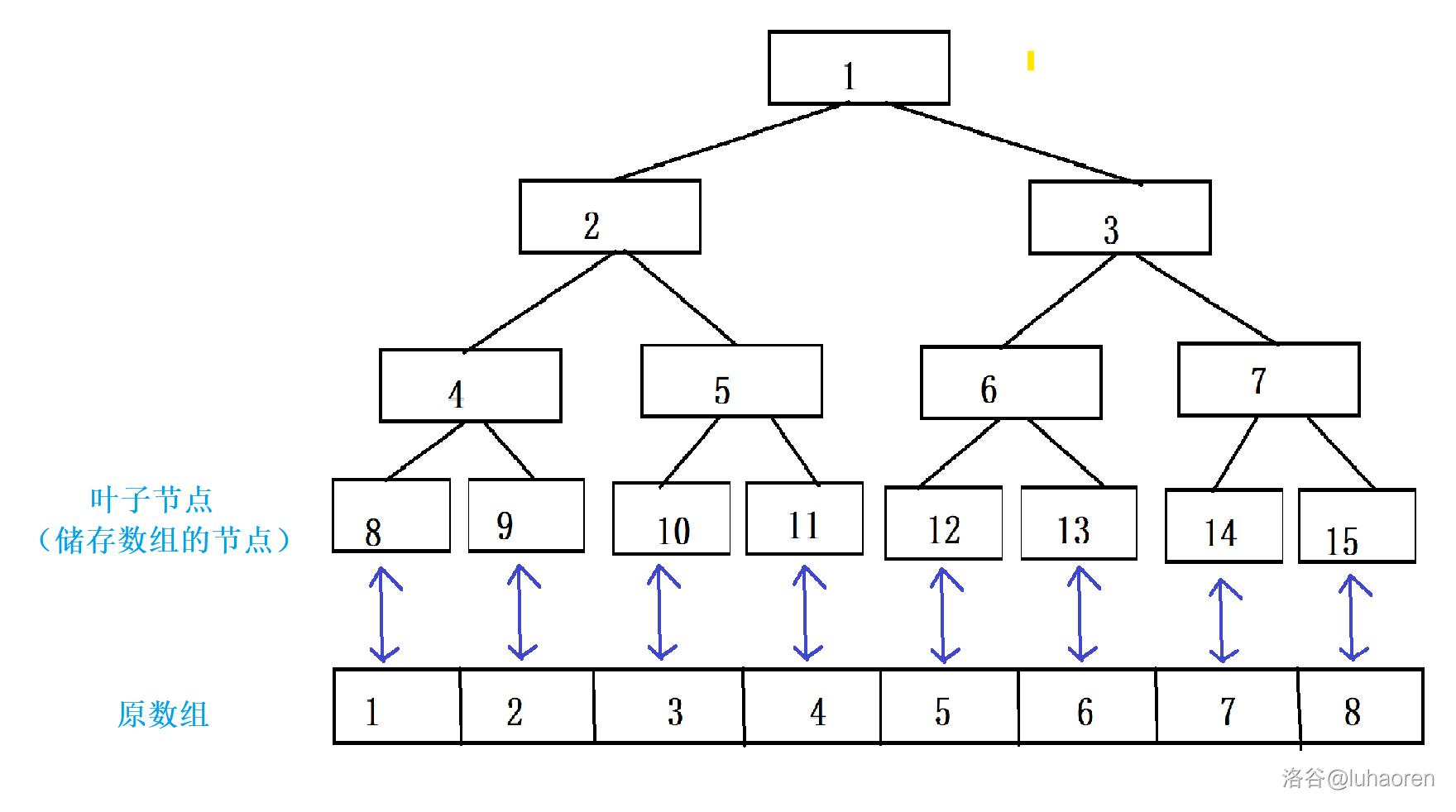int tr[N*4];//zkw線段樹不用維護子區間，直接開數組就行
int n,m;
void build(){
cin>>n>>m;
for(int i=n+1;i<=2*n;i++) cin>>tr[i];//直接讀入到葉子節點里
for(int i=n-1;i>=1;i--) tr[i]=tr[i*2]+tr[i*2+1];//自底向上更新
}


## 單點修改&區間查詢

### 單點修改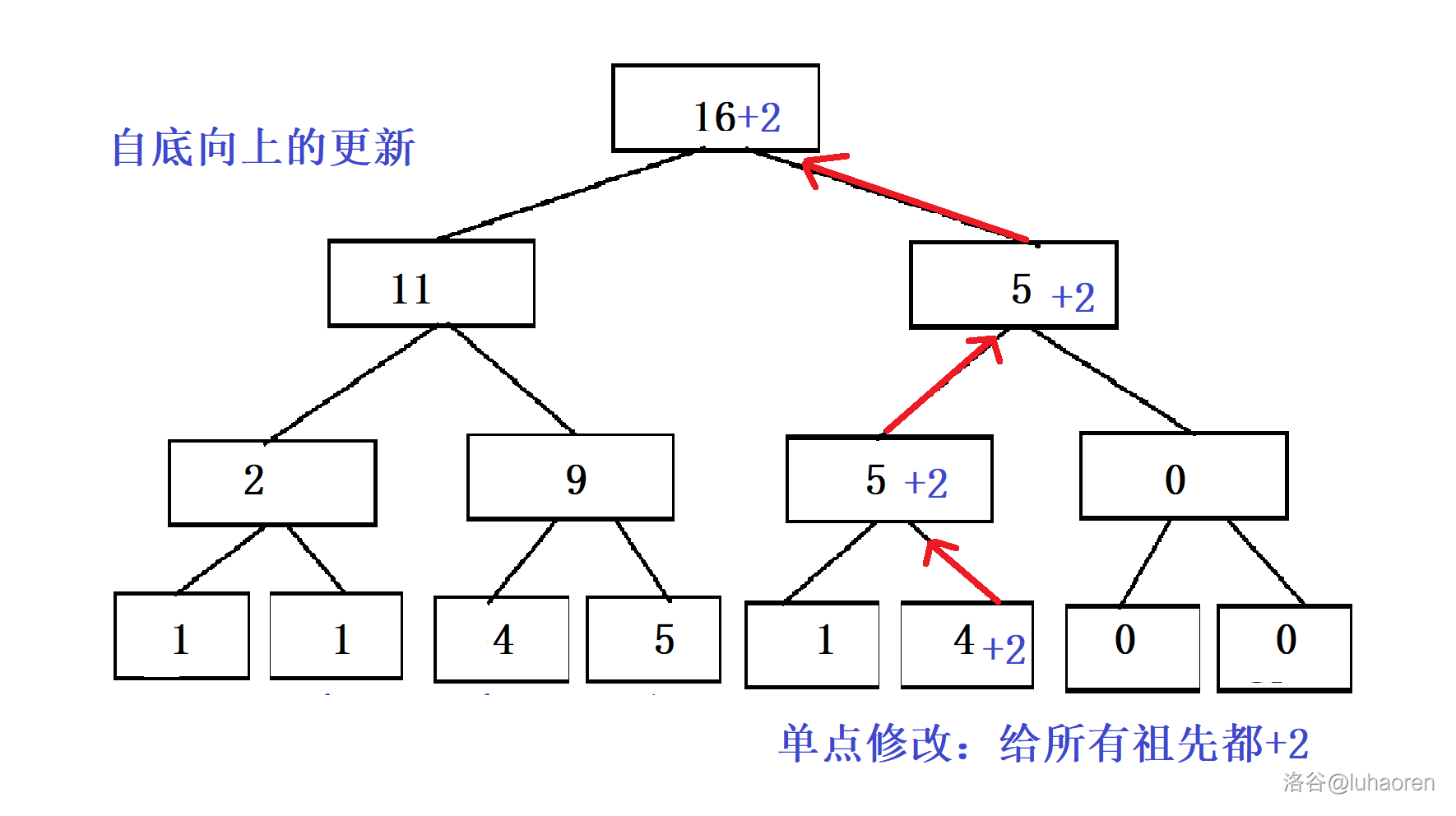inline void change(int x,int k){//給x加上k
for(int i=x+=n;i;i/=2) tr[i]+=k;//自底向上更新
}


inline int query_one(int x){//查詢x值
return tr[x+n];
}


### 區間查詢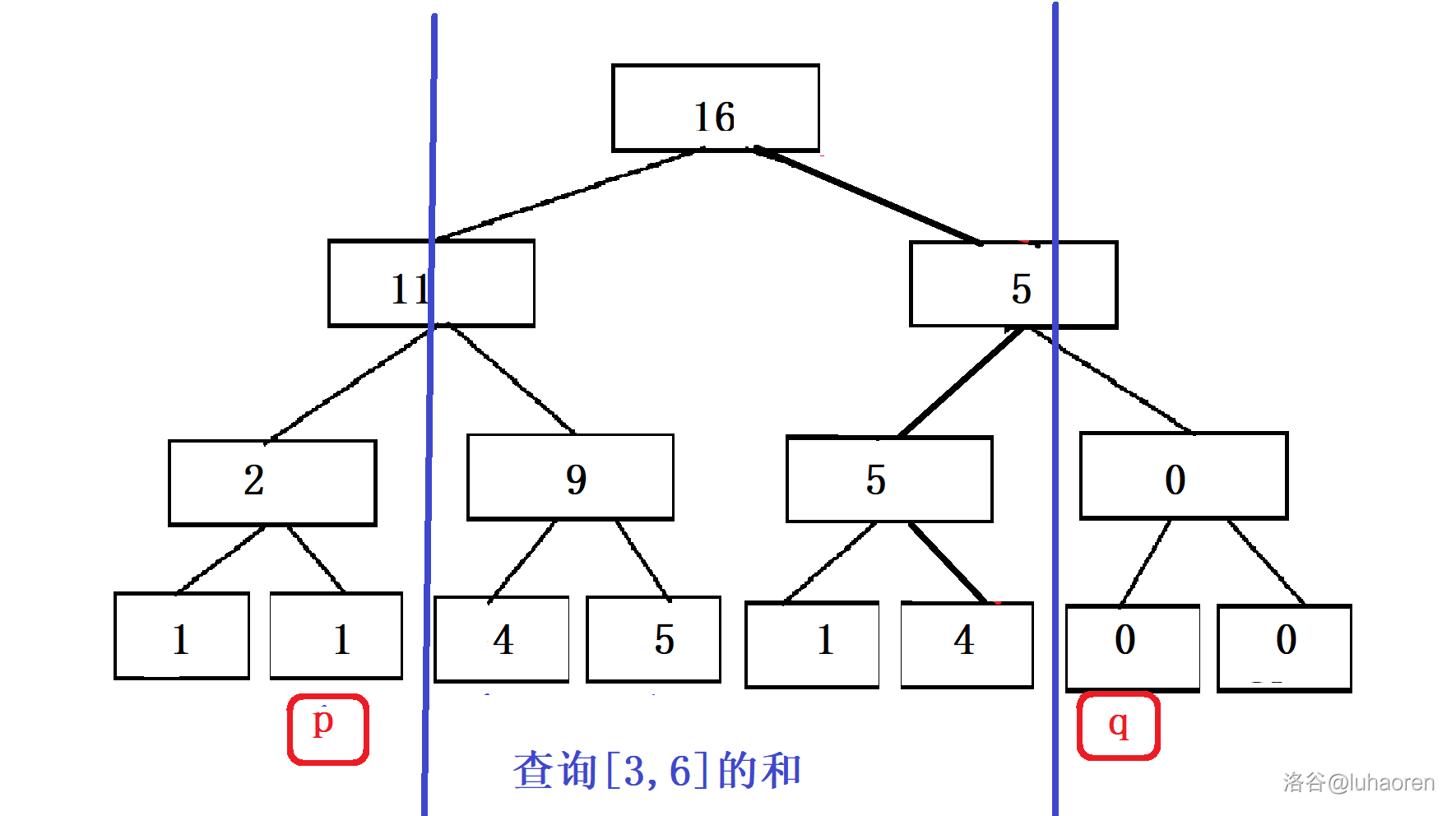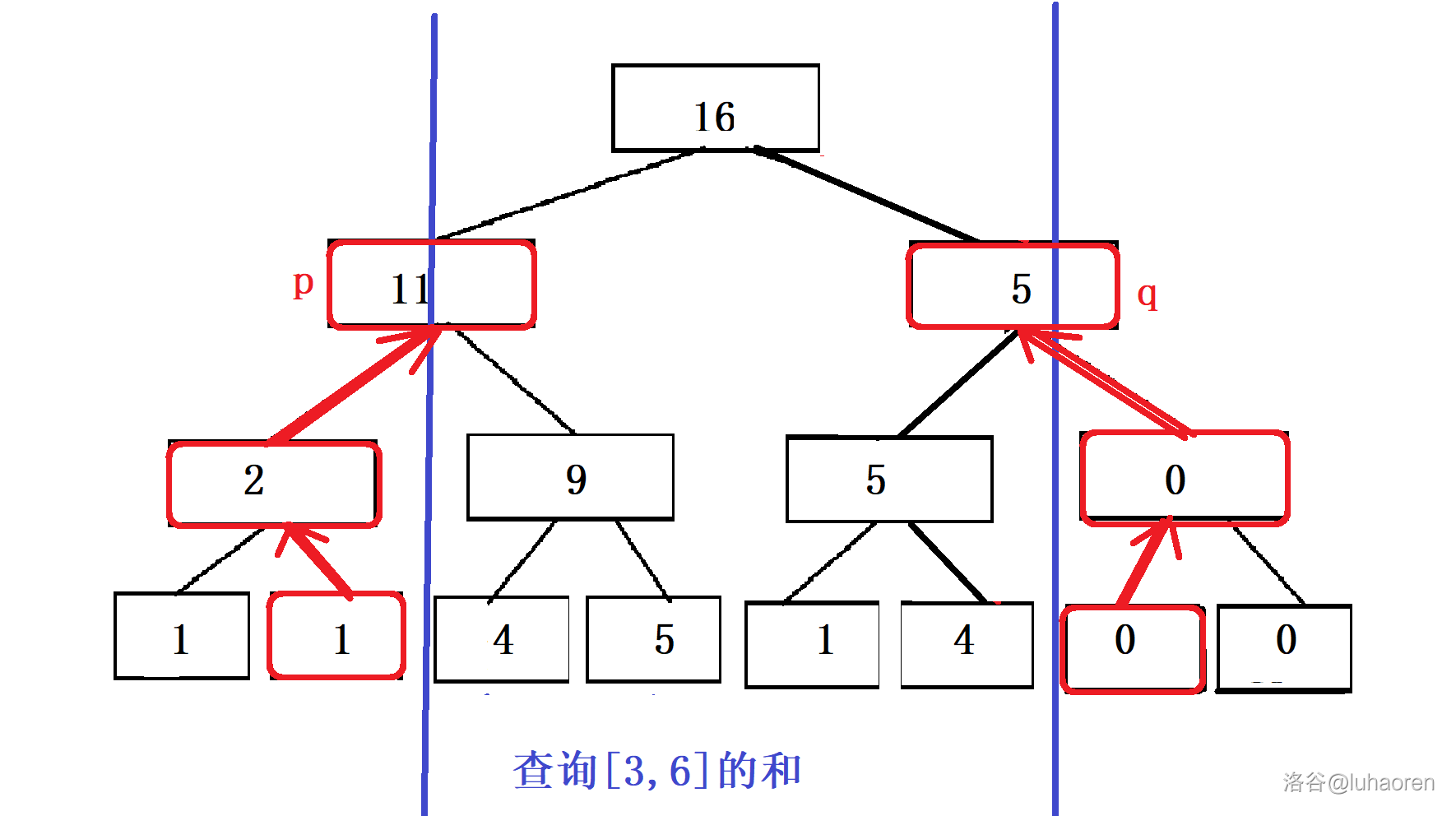1. $$p$$指向的節點是左兒子，那么答案加上右兒子的值

2. $$q$$指向的節點是右兒子，那么答案加上左兒子的值

inline int query(int l,int r){
int ans=0;
for(int p=l-1,q=r+1;p/2!=q/2;p/=2,q/=2){
if(!(p%2)) ans+=tr[p+1];//第一種情況
if(q%2) ans+=tr[q-1];//第二種情況
}
return ans;
}


### 習題

1. P3374 單點修改，區間查詢 用zkw線段樹再做一遍

## 區間修改&單點查詢

### 區間修改

zkw線段樹也支持區間修改，但是由于很難做到pushdown，所以zkw線段樹采用標記永久化的方式進行區間修改。

void uplate(int l,int r,int k){//給l,r區間內的數加上k
for(int p=l-1,q=r+1;p/2!=q/2;p/=2,q/=2){
}
}


### 單點查詢

inline int query_one(int x){//查詢x值
int sum=0;
}


1. P3372 線段樹1
用zkw線段樹做一遍
2. P3368 樹狀數組2
區間修改，單點查詢

# 可持久化線段樹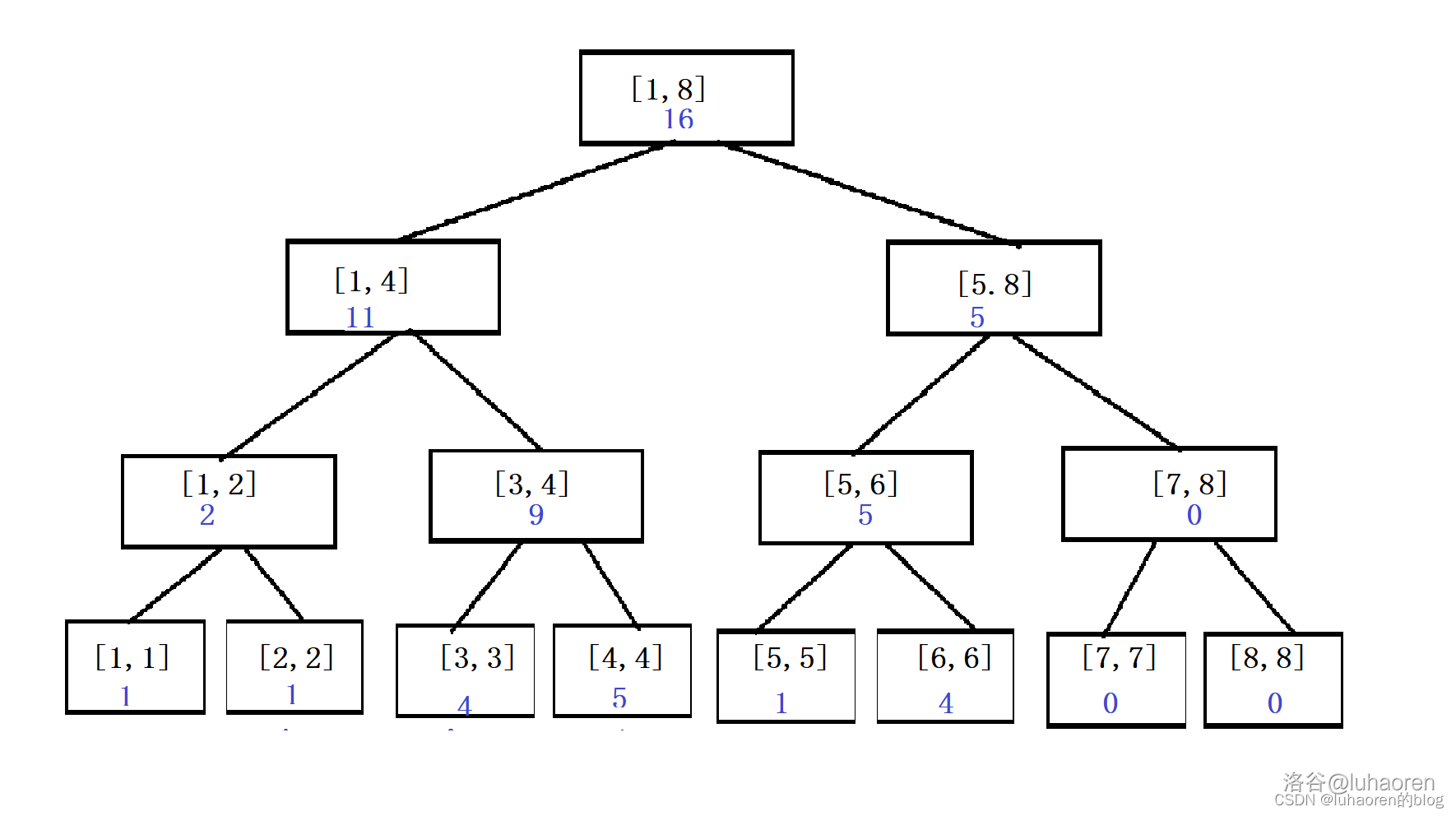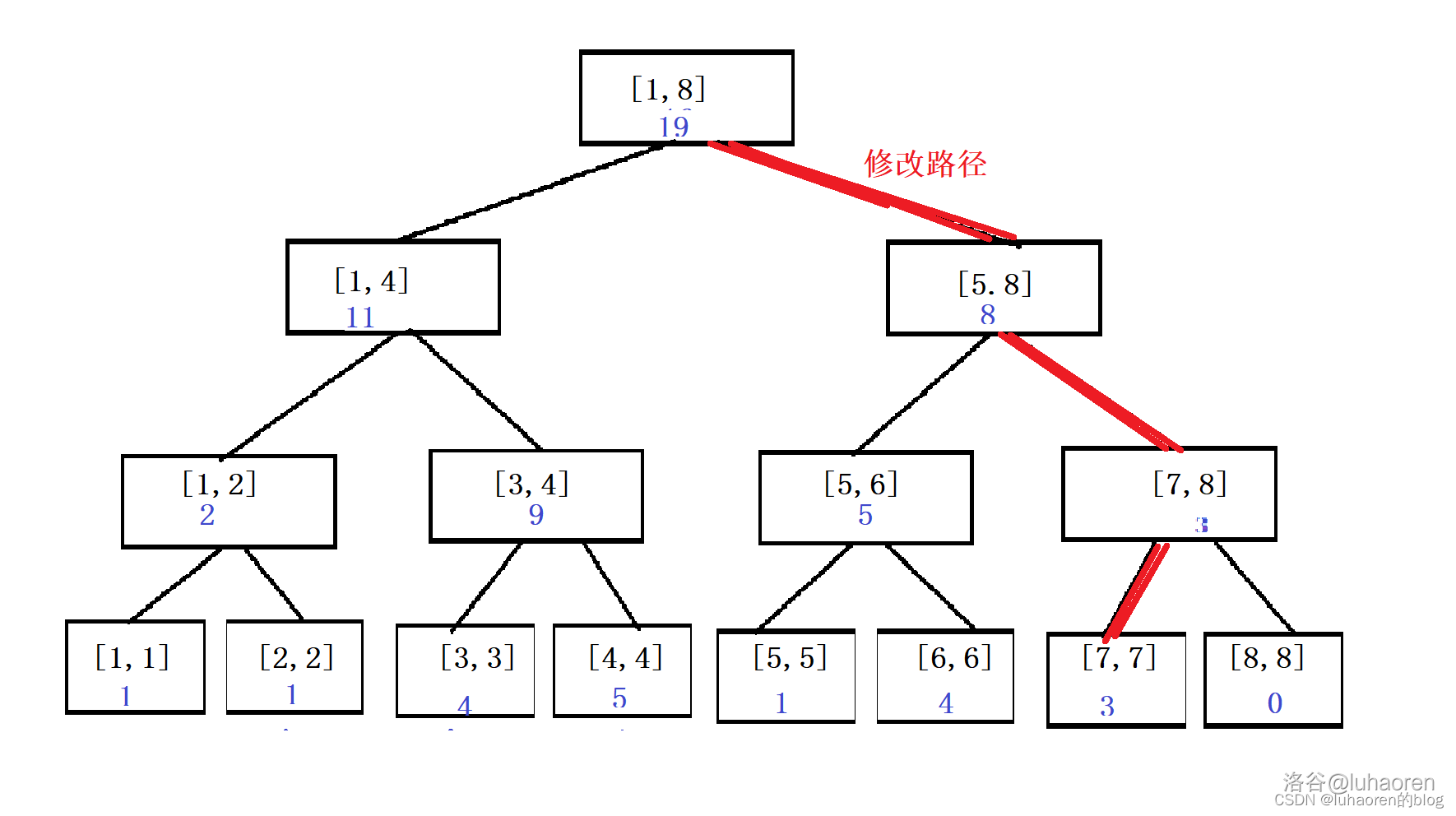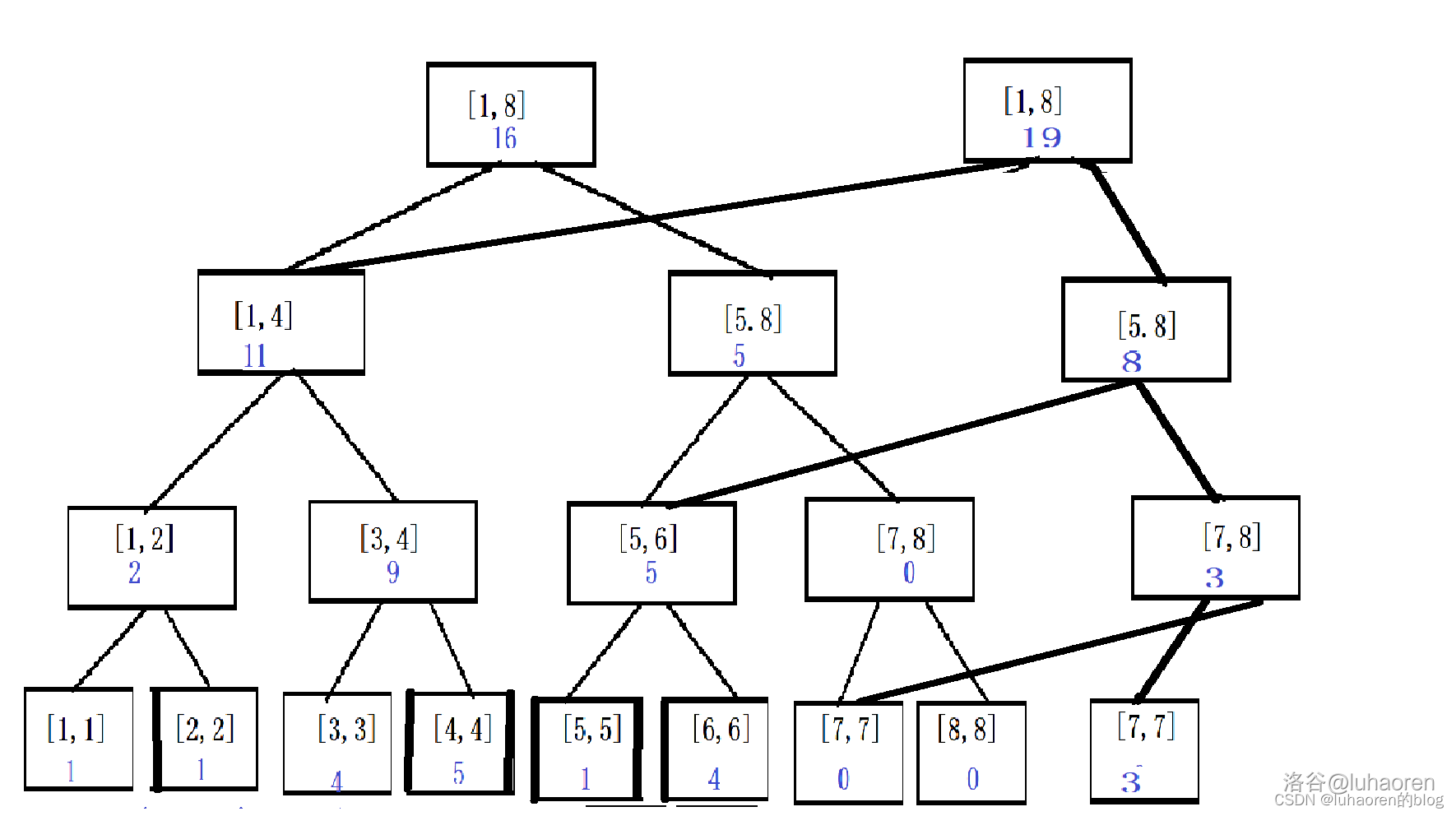void build(int &x,int l,int r){
//建樹操作，即第0個版本，所有版本復用的基礎
x=++tot;//可持久化線段樹使用動態開點的方式，因此需要有lsrs數組存儲左右兒子節點
if(l==r){
tr[x]=a[l];
return;
}
int mid=(l+r)/2;
build(ls[x],l,mid),build(rs[x],mid+1,r);
//因為x是引用形式，所以會直接給lsrs數組賦值
}
void change(int u,int &x,int l,int r,int k,int p){
x=++tot;
tr[x]=tr[u],ls[x]=ls[u],rs[x]=rs[u];
//復制原節點
if(l==r){
tr[x]=p;
return;
}
int mid=(l+r)/2;
if(k<=mid) change(ls[u],ls[x],l,mid,k,p);//修改左兒子，右兒子直接復用原節點的右兒子
else change(rs[u],rs[x],mid+1,r,k,p);//同理
}


### 例題6：可持久化數組

1. 在某個歷史版本上修改某一個位置上的值
2. 訪問某個歷史版本上的某一位置的值

AC代碼

#include <bits/stdc++.h>
using namespace std;
const int N=1e6+10,M=5e7+10;//可持久化線段樹大概需要O(4n+mlogn)的空間，一般直接開N<<5
int tr[M],root[N],ls[M],rs[M],tot=0,a[N];
void build(int &x,int l,int r){
x=++tot;
if(l==r){
tr[x]=a[l];
return;
}
int mid=(l+r)/2;
build(ls[x],l,mid),build(rs[x],mid+1,r);
}
void change(int u,int &x,int l,int r,int k,int p){
x=++tot;//動態開點
tr[x]=tr[u],ls[x]=ls[u],rs[x]=rs[u];//復制節點
if(l==r){
tr[x]=p;
return;
}
int mid=(l+r)/2;
if(k<=mid) change(ls[u],ls[x],l,mid,k,p);
else change(rs[u],rs[x],mid+1,r,k,p);
}
int query(int x,int l,int r,int k){
if(l==r) return tr[x];
int mid=(l+r)/2;
if(k<=mid) return query(ls[x],l,mid,k);
return query(rs[x],mid+1,r,k);
}
int n,m;
int main(){
scanf("%d%d",&n,&m);//本題稍微有點卡常，需要用printf和scanf
for(int i=1;i<=n;i++) scanf("%d",&a[i]);
build(root,1,n);
for(int i=1;i<=m;i++){
int v,opt,k,p;
scanf("%d%d",&v,&opt);
if(opt==1){
scanf("%d%d",&k,&p);
change(root[v],root[i],1,n,k,p);
}
else{
scanf("%d",&k);
printf("%d\n",query(root[v],1,n,k));
root[i]=root[v];
}
}
}


### 例題7：靜態區間第k小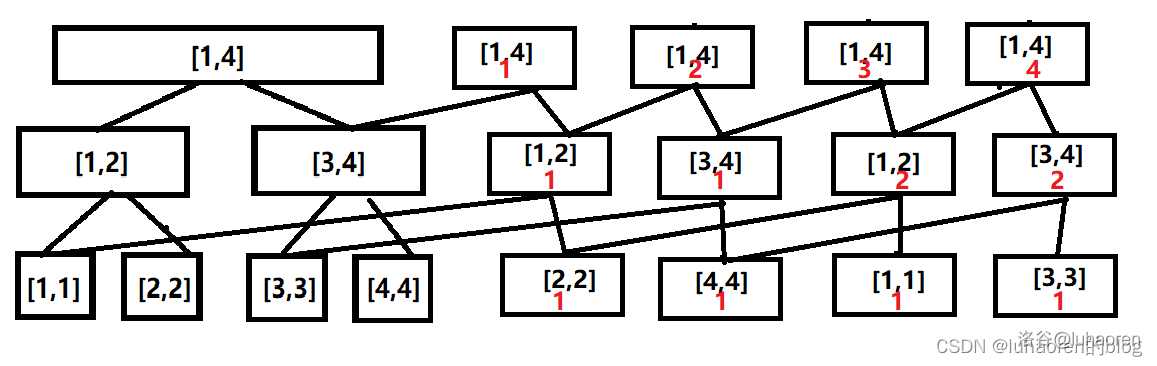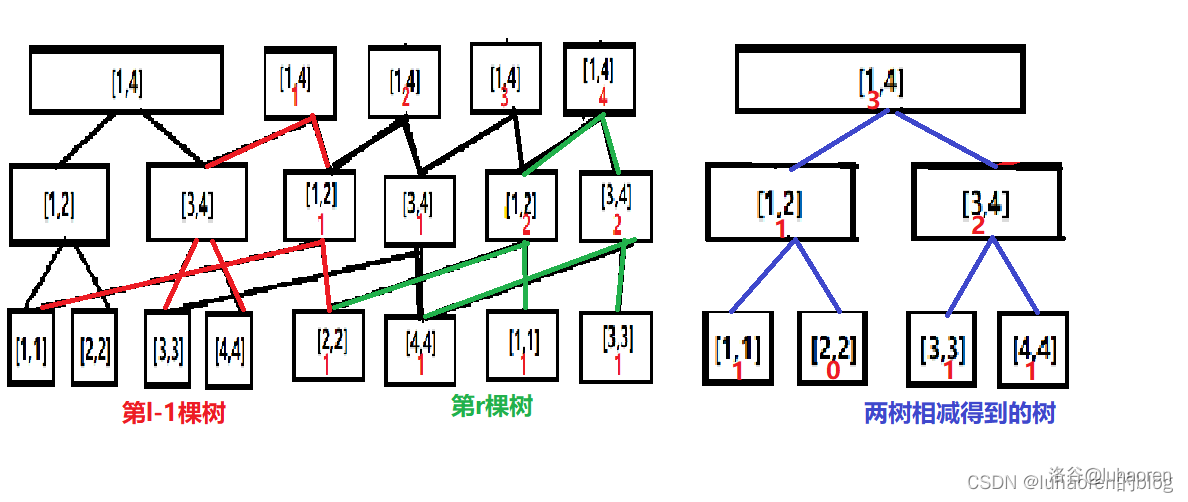AC代碼

#include <bits/stdc++.h>
using namespace std;
const int N=1e6+10;
int tr[N<<5],ls[N<<5],rs[N<<5],root[N],tot=0;
void build(int &x,int l,int r){
//建樹
x=++tot;
if(l==r) return;
int mid=(l+r)/2;
build(ls[x],l,mid),build(rs[x],mid+1,r);
}
void insert(int u,int &x,int l,int r,int k){
x=++tot;//動態開點
tr[x]=tr[u]+1,ls[x]=ls[u],rs[x]=rs[u];//復制該節點的所有信息（可以直接在節點上+1，否則還得pushuo一遍）
if(l==r)  return;
int mid=(l+r)/2;
if(k<=mid) insert(ls[u],ls[x],l,mid,k);
else insert(rs[u],rs[x],mid+1,r,k);
}
int query(int u,int v,int l,int r,int k){
int mid=(l+r)/2,lx=tr[ls[v]]-tr[ls[u]];//兩顆樹信息相減得到的左兒子信息
if(l==r) return l;//如果只有一個數，第幾大都是這個數了，直接返回
if(k<=lx) return query(ls[u],ls[v],l,mid,k);
return query(rs[u],rs[v],mid+1,r,k-lx);//二分查找第k小
}
int n,m;
int main(){
cin>>n>>m;
build(root,0,1e6);//建樹
for(int i=1;i<=n;i++){
int t;
cin>>t;
insert(root[i-1],root[i],0,1e6,t);
}
while(m--){
int l,r,k;
cin>>l>>r>>k;
cout<<query(root[l-1],root[r],0,1e6,k)<<endl;
}
}


//其他部分和前面無異，這以后是離散化代碼
int n,m,tt=0;
map<int,int>mp;//使用map離散化，使用sort離散化也可以
int val[N],a[N];
int main(){
cin>>n>>m;
build(root,1,n);
for(int i=1;i<=n;i++){
cin>>a[i];
mp[a[i]]=0;
}
for(auto it:mp){
//map會自己排序，在遍歷的過程中標上映射后的序號
mp[it.first]=++tt;
val[tt]=it.first;
}
for(int i=1;i<=n;i++) insert(root[i-1],root[i],1,n,mp[a[i]]);
while(m--){
int l,r,k;
cin>>l>>r>>k;
cout<<val[query(root[l-1],root[r],1,n,k)]<<endl;
}
}


### 習題

1. 洛谷P3402 可持久化并查集
注意并查集的合并操作
2. [POI2014] KUR-Couriers
維護區間絕對眾數，有亂搞做法

# U.P.D

2023年2月17日 初稿，大概兩千多字。

2023年6月？ cry拿去學，發現了一堆錯誤（比如代碼寫了個tr[x]=tr[x*2]+tr[x*2]）。

2023年7月3日 開始重寫。

2023年7月6日 寫完基礎部分

2023年7月8日 增加了權值線段樹

2023年7月9日 挪到了洛谷上，把圖片傳到了洛谷圖床上。增加了權值線段樹的習題。

2023年7月9日 增加了zkw線段樹

2023年7月11日 增加了可持久化線段樹

# 參考資料

posted @ 2023-10-23 18:05  烈風光翼  閱讀(133)  評論(0編輯  收藏  舉報